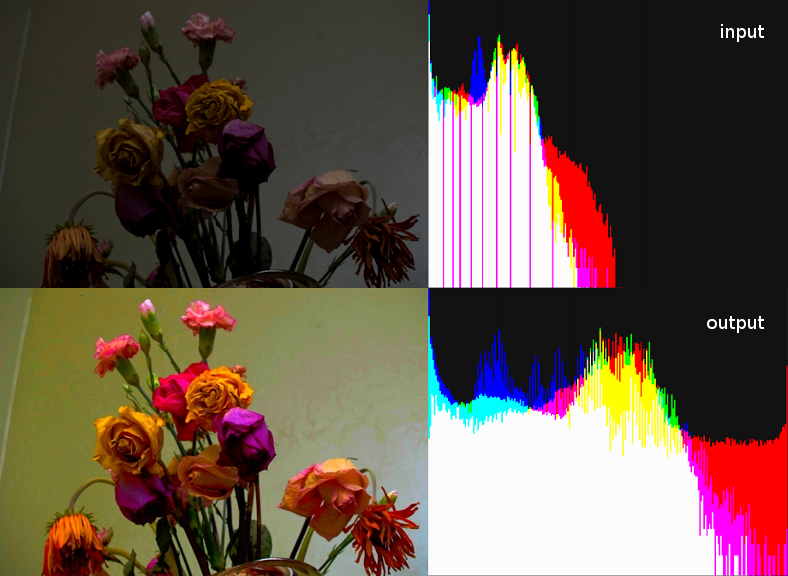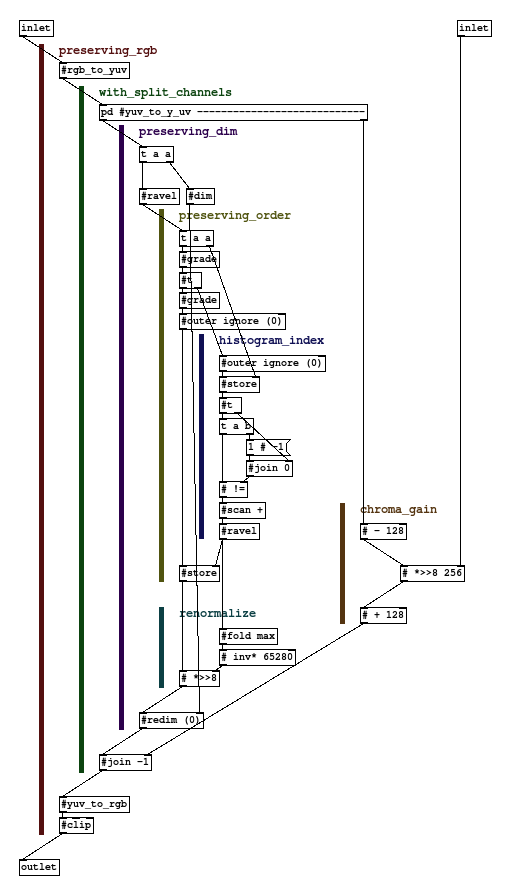# mathr / blog / #

## Histogram equalizationI first implemented equalization for Mandelbrot Set normalized iteration counts, which went something along these lines:

/* compare with neighbours too */
int float_cmpx(const void *a, const void *b) {
const float *x = a;
const float *y = b;
if (*y <= *x && *x < *(y+1)) return 0;
if (*x < *y) return -1;
if (*y < *x) return  1;
return 0;
}

/* equalize escapees to lie evenly in (0..1] */
void equalize(float *dwell, int n) {
/* make a copy with extra end elements */
float *dwellcopy = calloc(1, (n + 2) * sizeof(float));
memcpy(dwellcopy + 1, dwell, n * sizeof(float));
/* sort the valid part of the copy */
qsort(dwellcopy + 1, n, sizeof(float), float_cmp);
dwellcopy = -1e30;
dwellcopy[n+1] = 1e30;
/* ignore non-escaped points */
int mink = 0;
for (int k = 0; k < n; ++k) {
if (dwellcopy[k+1] <= 0) { ++mink; } else { break; }
}
/* get normalized index */
#pragma omp parallel for schedule(static, 1)
for (int j = 0; j < n; ++j) {
float d = dwell[j];
if (d <= 0) { continue; }
float *e = bsearch(&d, dwellcopy, n+1, sizeof(float), float_cmpx);
dwell[j] = ((1 + e - dwellcopy - mink) / (double) (1 + n - mink);
}
/* no longer need the copy */
free(dwellcopy);
}

Note: float_cmpx() and the extra padding elements are unnecessary in this particular snippet because it uses the whole image as control source: my real code uses only part of the image as control while equalizing the whole image, this means bsearch() might return NULL if using a normal comparator: the neighbour comparator will always succeed assuming the extra padding elements are outside the range of the entire input.

This worked out quite well for the floating point images I was using as input, which had a very low probability of multiple pixels having the same value. But when I tried to apply the same sort/lookup technique to regular images with only 8 bits per channel, the collision likelihood is so much greater that this simple technique fails.

The solution is to take into account collisions, and collapse the (possibly wide) range of indices for identical values down to 1 unit. I implemented this in GridFlow for Pure-data like this:The output image above is with the chroma gain set to 1536. Notice how the peaks in the output histogram are wider and the troughs shallower. The input image is from quick tricks for correcting poor exposure.